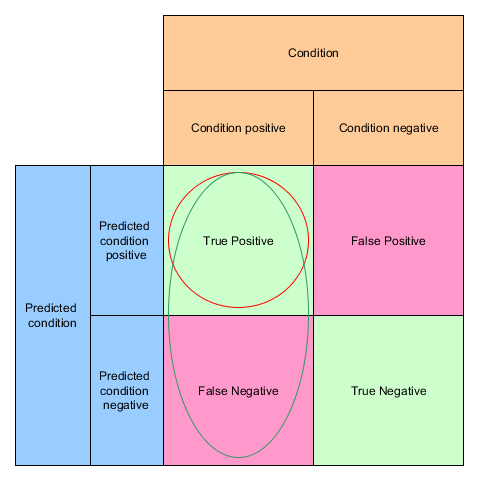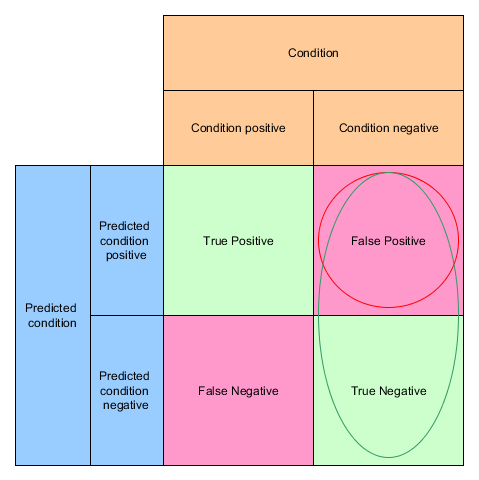## ROC Curve

As we already introduced Precision and Recall  the ROC curve is another way of looking at the quality of classification algorithms.

ROC stands for Receiver Operating Characteristic

The ROC curve is created by plotting the true positive rate (TPR) on the y-axis against the false positive rate (FPR) on the x-axis at various threshold settings.

You already know the TPR as recall or sensitivity.The false positive rate is defined as FPR = FP / (FP + TN)`from sklearn.metrics import roc_curve`